CBSE Class 10 Sample Paper for 2022 Boards - Maths Standard [Term 2]

Class 10
Solutions of Sample Papers for Class 10 Boards

## Two vertical poles of different heights are standing 20m away from each other on the level ground. The angle of elevation of the top of the first pole from the foot of the second pole is 60° and angle of elevation of the top of the second pole from the foot of the first pole is 30°. Find the difference between the heights of two poles. (Take √3 = 1.73)

This question is similar to Ex 9.1, 9 - Chapter 9 Class 10 - Some Applications of Trignometry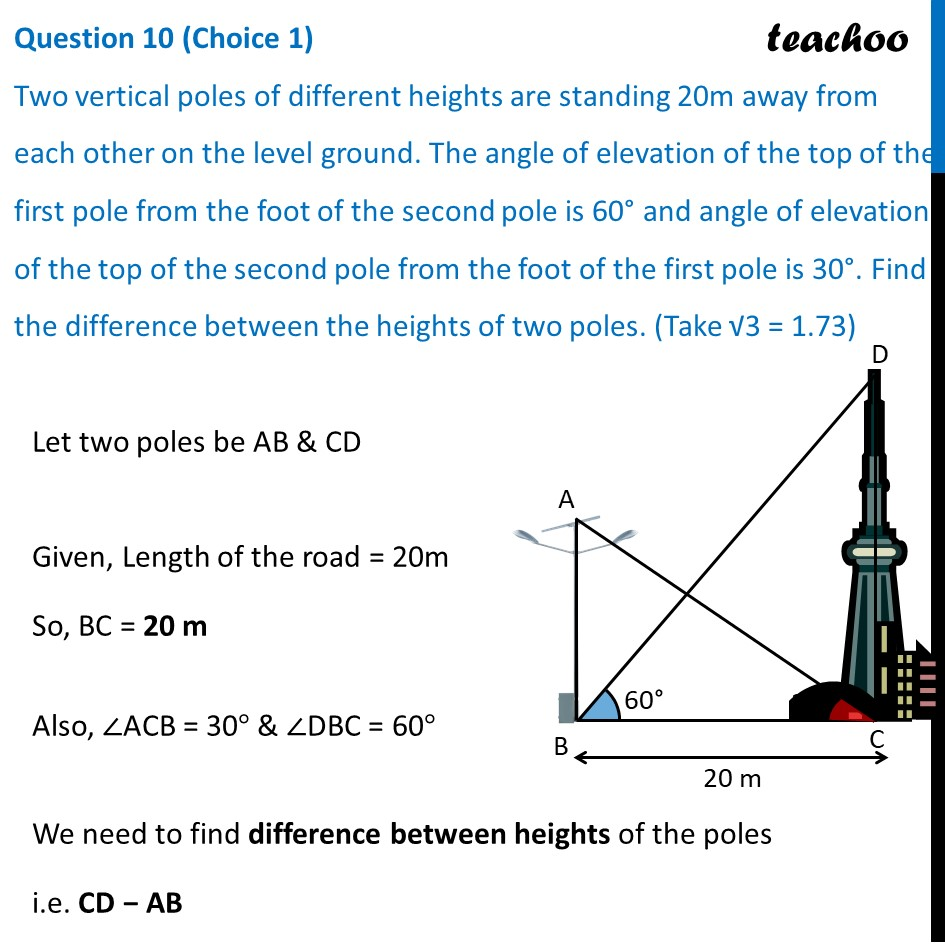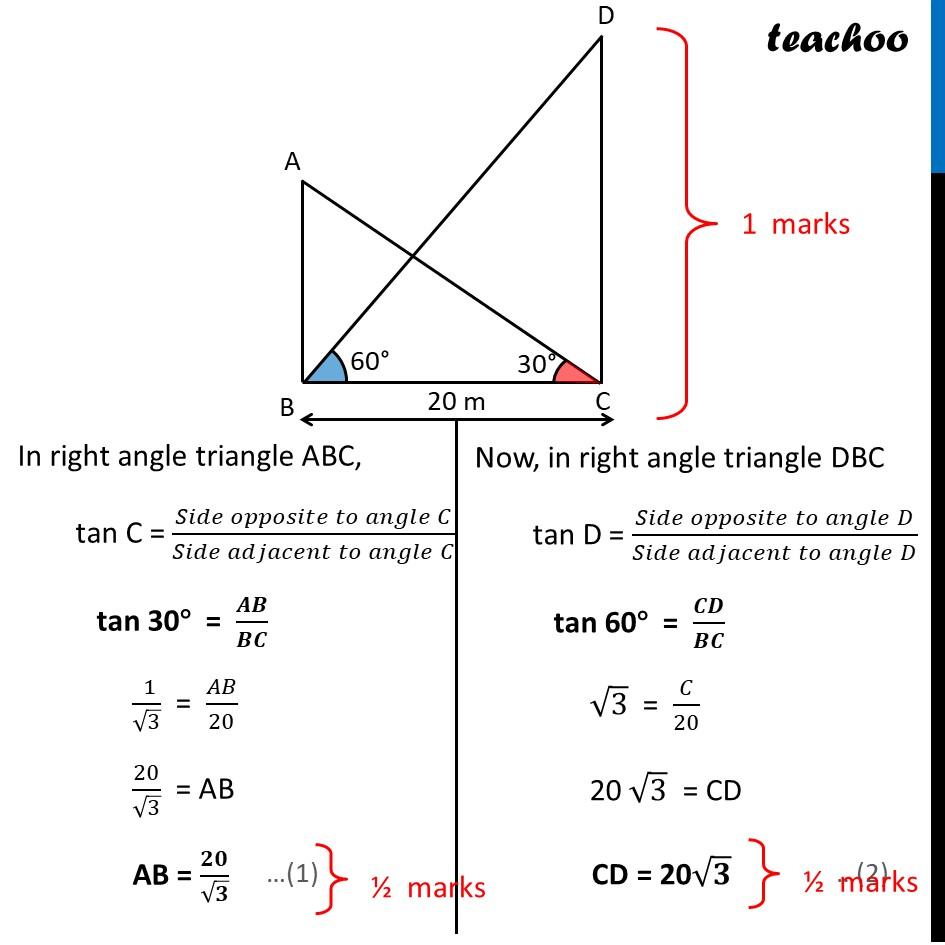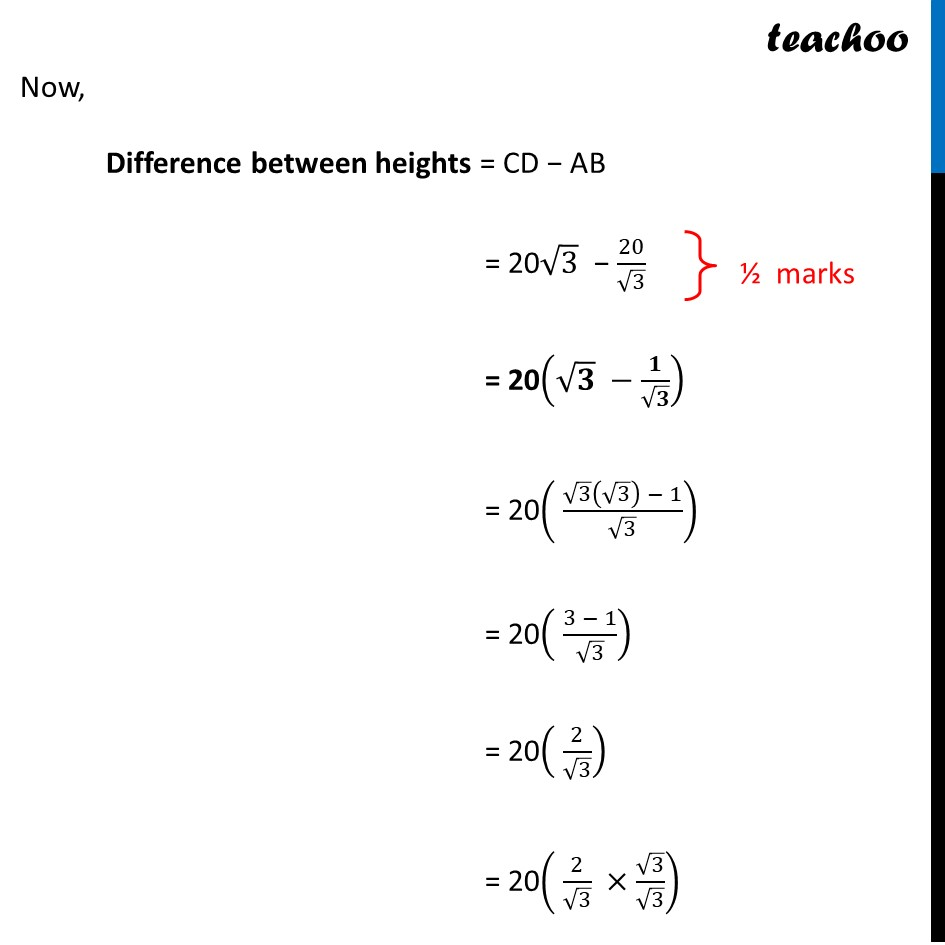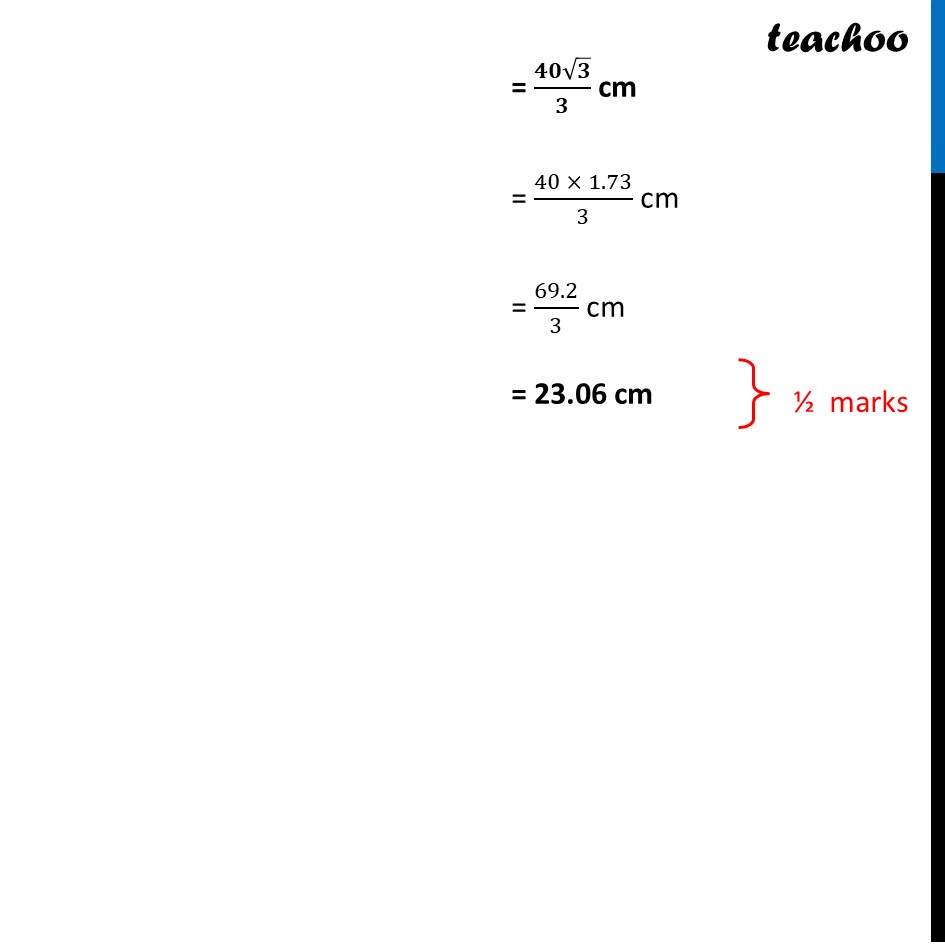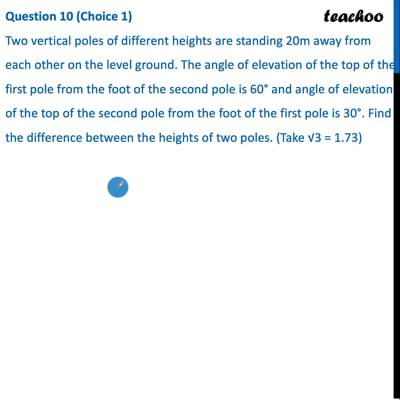This video is only available for Teachoo black users

### Transcript

Question 10 (Choice 1) Two vertical poles of different heights are standing 20m away from each other on the level ground. The angle of elevation of the top of the first pole from the foot of the second pole is 60° and angle of elevation of the top of the second pole from the foot of the first pole is 30°. Find the difference between the heights of two poles. (Take √3 = 1.73) Let two poles be AB & CD Given, Length of the road = 20m So, BC = 20 m Also, ∠ACB = 30° & ∠DBC = 60° We need to find difference between heights of the poles i.e. CD − AB In right angle triangle ABC, tan C = (𝑆𝑖𝑑𝑒 𝑜𝑝𝑝𝑜𝑠𝑖𝑡𝑒 𝑡𝑜 𝑎𝑛𝑔𝑙𝑒" " 𝐶)/(𝑆𝑖𝑑𝑒 𝑎𝑑𝑗𝑎𝑐𝑒𝑛𝑡 𝑡𝑜 𝑎𝑛𝑔𝑙𝑒" " 𝐶) tan 30° = 𝑨𝑩/𝑩𝑪 (" " 1)/√3 = 𝐴𝐵/20 20/√3 = AB AB = 𝟐𝟎/√𝟑 Now, in right angle triangle DBC tan D = (𝑆𝑖𝑑𝑒 𝑜𝑝𝑝𝑜𝑠𝑖𝑡𝑒 𝑡𝑜 𝑎𝑛𝑔𝑙𝑒" " 𝐷)/(𝑆𝑖𝑑𝑒 𝑎𝑑𝑗𝑎𝑐𝑒𝑛𝑡 𝑡𝑜 𝑎𝑛𝑔𝑙𝑒" " 𝐷) tan 60° = 𝑪𝑫/𝑩𝑪 √3 = 𝐶/20 20 √3 = CD CD = 20√𝟑 Now, Difference between heights = CD − AB = 20√3 − 20/√3 = 20(√𝟑 −𝟏/√𝟑) = 20( (√3 (√3) − 1)/√3) = 20( (3 − 1)/√3) = 20( 2/√3) = 20( 2/√3 ×√3/√3) = (𝟒𝟎√𝟑)/𝟑 cm = (40 × 1.73)/3 cm = 69.2/3 cm = 23.06 cm